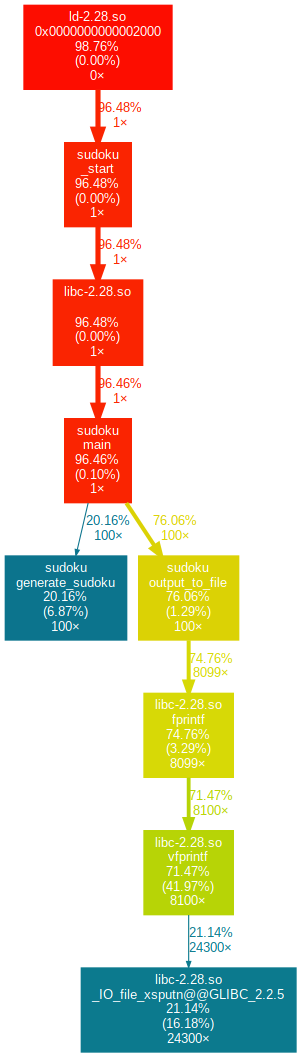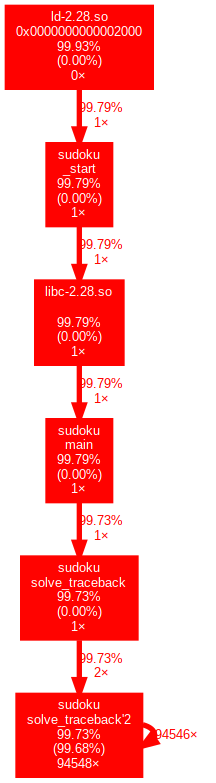# 一个简单的数独

1. 交换两行

2. 交换两列

3. 大型块交换

4. 矩阵翻转

5. 数字交换

## 代码分析

### 生成数独

    int mat_num = rand() % 4;
Sudoku rt;
// init
for(int i = 0; i < 9; i++) for(int j = 0; j < 9; j++)
rt.sudoku[i][j] = init_matrix[mat_num][i][j];
// shift line
for(int i = 0; i < 20; i++){
int block_num = rand() % 3; //随机选择一个“块”
int first_shift = rand() % 3;
int second_shift = rand() % 2;
if(second_shift >= first_shift) second_shift ++; //随机选择要交换的两行
first_shift += block_num * 3;
second_shift += block_num * 3; //与块相加
int tmp;
for(int i = 0; i < 9; i++){
tmp = rt.sudoku[first_shift][i];
rt.sudoku[first_shift][i] = rt.sudoku[second_shift][i];
rt.sudoku[second_shift][i] = tmp;
} //交换两行
}
//shift column
for(int i = 0; i < 20; i++){
int block_num = rand() % 3; //选择一个块
int first_shift = rand() % 3;
int second_shift = rand() % 2;
if(second_shift >= first_shift) second_shift ++;
first_shift += block_num * 3;
second_shift += block_num * 3; //选择要交换的两列
int tmp;
for(int i = 0; i < 9; i++){
tmp = rt.sudoku[i][first_shift];
rt.sudoku[i][first_shift] = rt.sudoku[i][second_shift];
rt.sudoku[i][second_shift] = tmp;
} //交换两列
}


### 求解数独

void solve_traceback(Sudoku * s){
if(_sudoku_solve) return; //如果已经解决就直接退出
int _cnt = 0;
for(int i = 0; i < 9; i++) for(int j = 0; j < 9; j++)
if(s->sudoku[i][j]) _cnt ++;
if(_cnt != s->filled) error=1;
if(s->filled == 81){ //如果填满就打印数独，并且设置为已解决
_sudoku_solve = 1;
print_sudoku(s);
return;
}
//find the empty point
int i, j = 0;
_find_empty(s, &i, &j); //寻找空白点
s->filled++;
for(int num = 1; num < 10; num++){
int flag = check_one(s, i, j, num);
if(flag){
s->sudoku[i][j] = num;
solve_traceback(s);
}
}
//traceback
s->filled--;
//s->filled -= fill_num;
s->sudoku[i][j] = 0;
//for(i = 0; i < fill_num; i++)
//    s->sudoku[fill_x[i]][fill_y[i]] = 0;
}


## 实现过程

#ifndef _SUDOKU_STRUCT
#define _SUDOKU_STRUCT
typedef struct{
int sudoku;
int filled;
}Sudoku;
#endif


Sudoku数据结构由两部分组成，一部分是9*9的数独矩阵，另一部分是已填的数据，这个数据在回溯求解的时候会用得上。

* 将数独输出到文件
int output_to_file(FILE* file, Sudoku s);
* 从文件中读取数独
int load_from_file(FILE* file, Sudoku * s);
* 生成数独
Sudoku generate_sudoku();
* 检查数独是否合法
int check_sudoku(Sudoku* s);
* 检查某个位置是否能填上某个数字
int check_one(Sudoku *s, int pt_i, int pt_j);
* 回溯求解
void solve_traceback(Sudoku * s);
* 输出数独
`void print_sudoku(Sudoku * s);“

## 性能分析

### 函数调用分析## PSP表格

PSP2.1 Personal Software Progress Stages 预估耗时（分钟） 实际耗时（分钟）
Planning 计划 45 30
Estimate 估计这个任务需要多少时间 2500 1500
Development 开发 1500 1800
Analysis 需求分析（包括学习新技术） 500 600
Design Spec 生成设计文档 60 60
Design Review 设计复审（和同事审核设计文档） 30 15
Coding Standard 代码规范（为目前的开发制定合适的规范） 30 20
Design 具体设计 200 300
Coding 具体编码 600 450
Code Review 代码复审 30 35
Test 测试（自我测试、修改代码、提交测试） 200 300
Reporting 报告 20 60
Test Report 测试报告 20 20
Size Measurement 计算工作量 10 10
Postmortem & Process Improvement Plan 事后总结，并提出过程改进计划 60 30